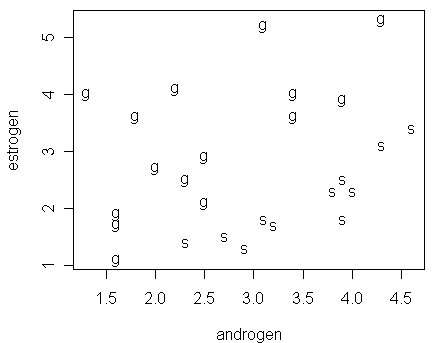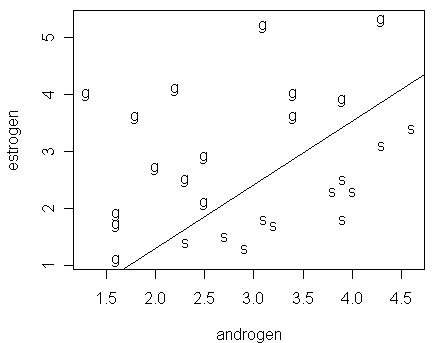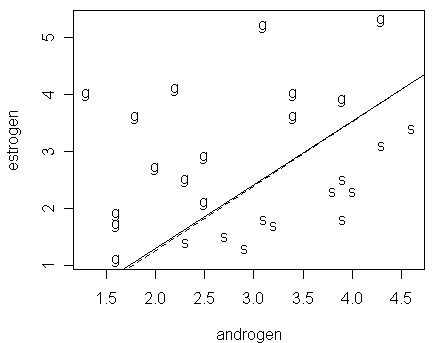Estimation Problem (Reading: Faraway (2006), section 2.8)

Margolese (1970) reported a data that recorded urinary androsterone (androgen) and etiocholanolone (estrogen) values from 26 healthy males. Among them, 15 men are homosexual (labeled as "g" in the orientation variable) and 11 heterosexual (labeled as "s"). We start by reading the data into R and drawing a plot of it:

> hormone

androgen estrogen orientation

1       3.9      1.8           s

2       4.0      2.3           s

3       3.8      2.3           s

…deleted…

26      1.3      4.0           g

> plot(estrogen ~ androgen,data=hormone,pch=as.character(orientation))Notice that:

• it seems possible to draw a clear-cut line in the figure to separate the two groups of men

• this is an example of "sparse data," i.e., data with small nx (nx=1 in this case)

• Q: for sparse data, what analysis results of binomial GLM will become less reliable?

We now fit a binomial GLM to see if the orientation can be predicted from the two hormone values:

> modl <- glm(orientation ~ estrogen + androgen, hormone, family=binomial)

Warning messages:

1: algorithm did not converge in: glm.fit(x = X, y = Y, weights = weights, start = start, etastart = etastart,

2: fitted probabilities numerically 0 or 1 occurred in: glm.fit(x = X, y = Y, weights = weights, start = start, etastart = etastart,

Notice that:

• when yx is a Bernoulli response (i.e, yx=0 or 1), we can put yx directly as the response variable in the glm function. There is no need to form another column that contains 1-yx

• as shown in the warning message, there were problems with the convergence of the algorithm used to approximate MLE

A look at the summary reveals further evidence:

> summary(modl)

Call:

glm(formula = orientation ~ estrogen + androgen, family = binomial, data = hormone)

Deviance Residuals:

Min          1Q      Median          3Q         Max

-2.759e-05  -2.107e-08  -2.107e-08   2.107e-08   3.380e-05

Coefficients:

Estimate Std. Error z value Pr(>|z|)

(Intercept)    -84.49  136095.03  -0.001        1

estrogen       -90.22   75910.98  -0.001        1

androgen       100.91   92755.62   0.001        1

(Dispersion parameter for binomial family taken to be 1)

Null deviance: 3.5426e+01  on 25  degrees of freedom

Residual deviance: 2.3229e-09  on 23  degrees of freedom

AIC: 6

Number of Fisher Scoring iterations: 25

Notice that

• the residual deviance is extremely small, which indicates that this is a very good fit

• however, chi-square goodness-of-fit test may not be accurate because of sparseness

• none of the predictors are significant under the Wald test; the insignificance is caused by their high standard errors

• two possible explanations of the high standard error: linearly separable property existing in the dataset as will be explained below and sparseness

• note: for sparse data, the standard errors may be overestimated Þ this is known as the Hauck-Donner effect Þ deviance-based test is preferred (see its results in the next command)

• the maximum default number of iterations (25) has been reached but the algorithm had not converged

• it implies the current estimated values of b are not reliable

• note: the convergence problem is caused by the linearly separable property, rather than sparseness

Let us now perform deviance-based method to test the significance of individual parameters:

> drop1(modl,test="Chi")

Single term deletions

Model:

orientation ~ estrogen + androgen

Df  Deviance    AIC    LRT   Pr(Chi)

<none>      2.323e-09  6.000

estrogen  1    27.437 31.437 27.437 1.623e-07 ***

androgen  1    28.257 32.257 28.257 1.062e-07 ***

---

Signif. codes:  0 '***' 0.001 '**' 0.01 '*' 0.05 '.' 0.1 ' ' 1

Notice that:

• both predictors have significant contribution to the reduction of deviance

• the deviance-based test reveals the information we would expect from the next figure --- both estrogen and androgen are now significant

• remember that for sparse data, the test involving differences of deviances is more accurate than the goodness-of-fit test involving only a single deviance

We now go back to the problem of unstable parameter estimation. Let us take a look at a figure, which computes the line corresponding to px=1/2, where px is predicted using the fitted model modl. The figure reveals the reason for the instability in parameter estimation:

> abline(-84.49/90.22,100.91/90.22)Notice that:

• the two groups are linearly separable so that a perfect fit is possible

• for the data, we suffer from an "embarrassment of riches" --- a perfect fit is achievable (Þ suggest that perfect predictions could be possible), but the estimates of the parameters and their standard errors are unstable

Let us try to estimate the parameters by using a bias-reduction method proposed by Firth (1993). The estimates have the advantage of always being finite:

> library(brlr)
> modb <- brlr(orientation ~ estrogen + androgen, hormone, family=binomial)
> summary(modb)

Call:

brlr(formula = orientation ~ estrogen + androgen, data = hormone, family = binomial)

Coefficients:

Value   Std. Error t value

(Intercept) -3.6512  2.9110    -1.2543

estrogen    -3.5862  1.4989    -2.3925

androgen     4.0743  1.6212     2.5132

Deviance: 3.6993

Penalized deviance: 4.1837

Residual df: 23

Notice that:

• the estimates obtained from the bias-reduction method are quite different from that in the glm result

• under the bias-reduction method, the two predictors are significant (because their t-values are quite extreme compared with a t-distribution with 23 degrees of freedom), which we expect given the previous figure

By using the fitted model in the brlr result, we add the line corresponding to px=1/2:

> abline(-3.6512/3.5862,4.0743/3.5862,lty=2)Notice that:

• although the fit from the brlr command appears to be different from the glm result (judging from their estimated values of parameters), their fitted probabilities are effectively very close as we can see from the figure

• whether the data is effective depends on the purpose of analysis

• for prediction or classification purpose, this is a good dataset, but

• it is not so useful for the purpose of parameter estimation